Next: The variable table Up: Order Previous: Order   Contents

General principle

During the bisection process new boxes will be created and stored in the list. But we want to order these new boxes so that the procedure will consider first the most promising box. The ordering is based on an evaluation index, the new boxes being stored using an increasing order of the index (the box with the lowest index will be stored first). The flag Order indicate which index is used: indexMAXCONSTRAINTFUNCTION@MAX_CONSTRAINT_FUNCTION

• MAX_FUNCTION_ORDER: let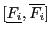be the interval evaluation of the optimum equation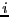. The index is obtained as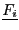for a minimization problem and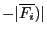for a maximization problem,
• MAX_CONSTRAINT_FUNCTION: same than MAX_FUNCTION_ORDER if there is only one equation in the system. Otherwise:
• if there are equality constraints use the MAX_FUNCTION_ORDER index
• if there are only inequality constraints and if they all hold for all the new boxes, then the index is the lower bound of the optimum function for a minimization problem and the upper bound for a maximization problem.
• if there are only inequality constraints and none of the new boxes satisfied them all: the index is the upper bound of the inequality for the constraint of type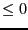and the absolute value of the lower bound for the constraint of type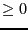• if there are only inequality constraints and they are all verified only for some of the new boxes, then the index will be calculated in such way that the boxes satisfying the constraints will be stored first according to the value of the lower or upper bound of the optimum function. Then will be stored the boxes not satisfying the constraints according to the index described in the previous item
• MAX_MIDDLE_FUNCTION_ORDER: let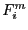be the value of the function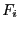computed for the middle point of the box. The index is the absolute value ofNext: The variable table Up: Order Previous: Order   Contents
Jean-Pierre Merlet 2012-12-20